# Yatzy Game

Learn creating a Yatzy Game using Windows App SDK with this Tutorial

Yatzy Game shows how to create a dice game based on Yacht or Yahtzee using a toolkit from NuGet using the Windows App SDK.

## Step 1

Follow Setup and Start on how to get Setup and Install what you need for Visual Studio 2022 and Windows App SDK.

In Windows 11 choose Start and then find or search for Visual Studio 2022 and then select it.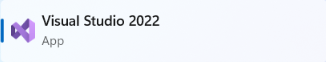Once Visual Studio 2022 has started select Create a new project.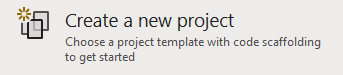Then choose the Blank App, Packages (WinUI in Desktop) and then select Next.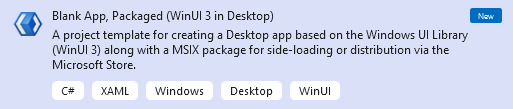After that in Configure your new project type in the Project name as YatzyGame, then select a Location and then select Create to start a new Solution.## Step 2

Then in Visual Studio within Solution Explorer for the Solution, right click on the Project shown below the Solution and then select Manage NuGet Packages...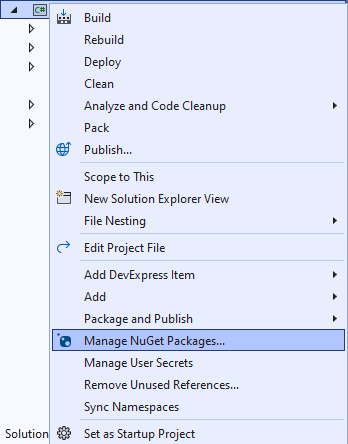## Step 3

Then in the NuGet Package Manager from the Browse tab search for Comentsys.Toolkit.WindowsAppSdk and then select Comentsys.Toolkit.WindowsAppSdk by Comentsys as indicated and select Install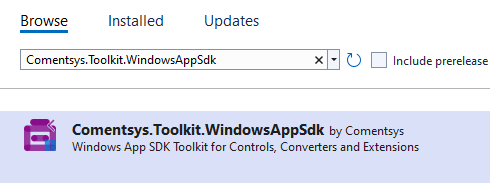This will add the package for Comentsys.Toolkit.WindowsAppSdk to your Project. If you get the Preview Changes screen saying Visual Studio is about to make changes to this solution. Click OK to proceed with the changes listed below. You can read the message and then select OK to Install the package, then you can close the tab for Nuget: YatzyGame by selecting the x next to it.

## Step 4

Then in Visual Studio within Solution Explorer for the Solution, right click on the Project shown below the Solution and then select Add then New Item…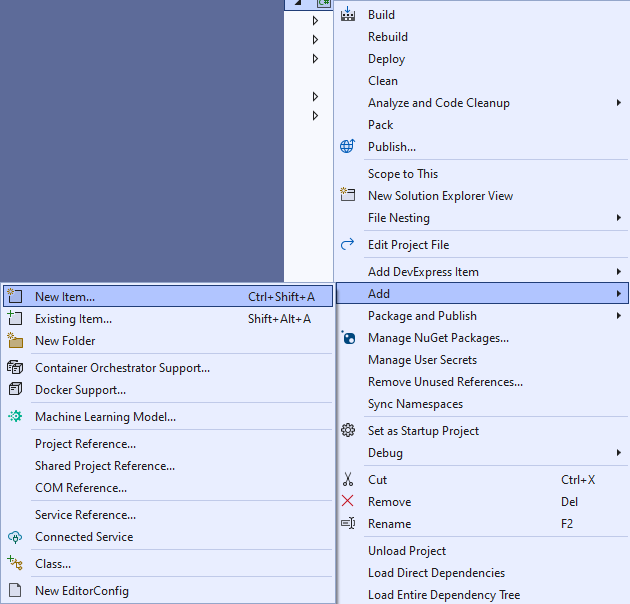## Step 5

Then in Add New Item from the C# Items list, select Code and then select Code File from the list next to this, then type in the name of Library.cs and then Click on Add.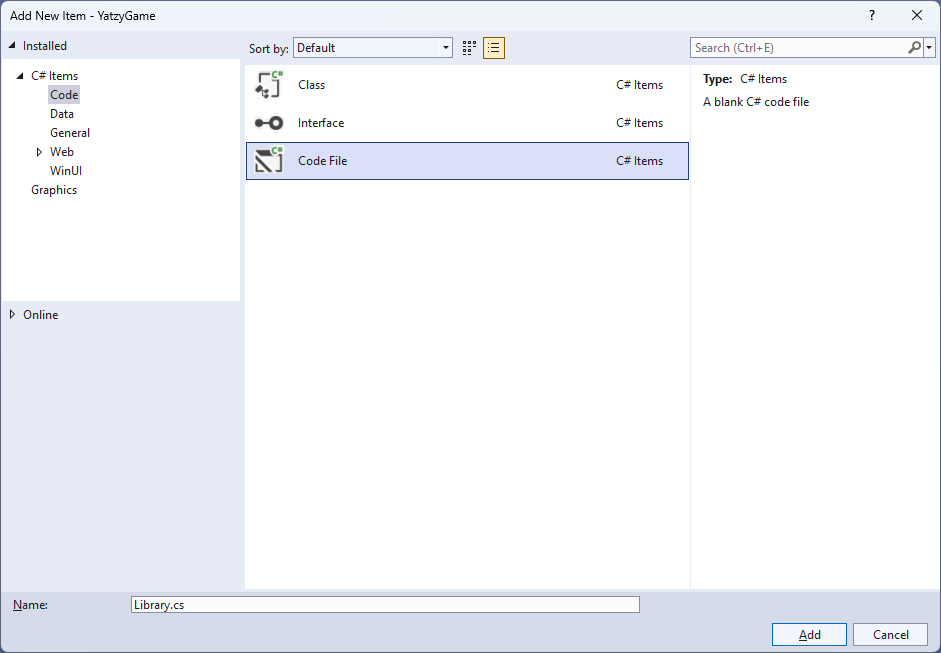## Step 6

You will now be in the View for the Code of Library.cs and then you need to type the following Code:

``````
// Usings & Namespace

public enum ScoreType
{
SixesScore, UpperTotalScore, UpperTotalBonusScore, ThreeOfAKindScore,
FourOfAKindScore, FullHouseScore, SmallStraightScore, LargeStraightScore,
YahtzeeScore, ChanceScore, YahtzeeBonusScore, LowerTotalScore, TotalScore
}

// Extensions Class

// Item Class

// Option Class

public class Calculate
{
// Calculate GetAddUp, GetOfAKind & GetFullHouse Method

// Calculate GetSmallStraight & GetLargeStraight Method

// Calculate Get GetYahtzee & GetChance Method

}

public class Board // : ActionCommandObservableBase
{
// Board Constants, Variables, Properties and Choose, SetScore & GetScore Method

// Board Clear & Reset Method

// Board SetTotal & AddUpDice Method

// Board SetValueOfAKind Method

// Board SetItemScore Method

// Board SetYahtzee Method

// Board SetChance, SetBonus & New Method

// Board Roll Method

// Board Constructor
}

// OptionTemplateSelector Class

// Library Class
``````

## Step 7

Still in Library.cs you will define a `namespace` which allows classes to be defined together, usually each is separate but will be defined in Library.cs along with adding `using` statements below the Comment of `// Usings & Namespace` by typing the following Code:

``````
using Comentsys.Toolkit.Binding;
using Comentsys.Toolkit.WindowsAppSdk;
using Microsoft.UI.Xaml;
using Microsoft.UI.Xaml.Controls;
using System;
using System.Collections.Generic;
using System.Linq;
using System.Text.RegularExpressions;

namespace YatzyGame;
``````

The `using` statements including ones for the package of `Comentsys.Toolkit.Binding` and `Comentsys.Toolkit.WindowsAppSdk` along with the `namespace` for `YatzyGame`.

## Step 8

Still in Library.cs for the `namespace` of `YatzyGame` you will define a `class` for `Extensions` after the Comment of `// Extensions Class` by typing the following:

``````
public static class Extensions
{
private const string space = " ";
private const string score = "Score";
private static readonly Regex regex = new(@"\p{Lu}\p{Ll}*");

public static string Name(this ScoreType type) =>
string.Join(space, regex
.Matches(Enum.GetName(typeof(ScoreType), type)
.Replace(score, string.Empty))
.Select(s => s.Value));

public static string Name(this ScoreType type, int take) =>
string.Join(space, regex
.Matches(Enum.GetName(typeof(ScoreType), type))
.Select(s => s.Value)
.Take(take));
}
``````

`Extensions` will provide formatting capabilities for outputting the values for elements in the game.

## Step 9

Still in Library.cs for the `namespace` of `YatzyGame` you will define a `class` for `Item` after the Comment of `// Item Class` by typing the following:

``````
public class Item : ActionCommandObservableBase
{
private int _value;
private bool _hold;

public int Index { get; }
public int Value { get => _value; set => SetProperty(ref _value, value); }
public bool Hold { get => _hold; set => SetProperty(ref _hold, value); }

public Item(int index, Action<int> action) :
base(new ActionCommandHandler((param) =>
action((param as Item).Index),
(param) => (param as Item).IsEnabled)) =>
(Index, Value) = (index, index + 1);
}
``````

`Item` has Properties for `Index`, `Value` and `State` plus uses `ActionCommandObservableBase` from the package of `Comentsys.Toolkit.WindowsAppSdk`.

## Step 10

Still in Library.cs for the `namespace` of `YatzyGame` you will define a `class` for `Option` after the Comment of `// Option Class` by typing the following:

``````
public class Option : ActionCommandObservableBase
{
private int _score;

public int Score { get => _score; set => SetProperty(ref _score, value); }
public ScoreType Type { get; }
public string Content => Type.Name();

public Option(ScoreType type) : base(null) =>
Type = type;

public Option(ScoreType type, Action<object> action) :
base(new ActionCommandHandler((param) =>
action(null),
(param) => (param as Option).IsEnabled)) =>
Type = type;
}
``````

`Option` has Properties for `Scoree`, `Type` and `Content` and also uses `ActionCommandObservableBase` from the package of `Comentsys.Toolkit.WindowsAppSdk`.

## Step 11

While still in the `namespace` of `YatzyGame` in Library.cs and in the `class` of `Calculate` after the Comment of `// Calculate GetAddUp, GetOfAKind & GetFullHouse Method` type the following Methods:

``````
public static int GetAddUp(Item[] dice, int value)
{
int sum = 0;
foreach(var item in dice.Where(w => w.Value == value))
sum += value;
return sum;
}

public static int GetOfAKind(Item[] dice, int value)
{
int sum = 0;
bool result = false;
for (int i = 1; i <= 6; i++)
{
int count = 0;
for (int j = 0; j < 5; j++)
{
if (dice[j].Value == i)
count++;
if (count > value)
result = true;
}
}
if(result)
{
foreach (var item in dice)
sum += item.Value;
}
return sum;
}

public static int GetFullHouse(Item[] dice)
{
int sum = 0;
int[] item = dice.Select(s => s.Value).ToArray();
Array.Sort(item);
if (((item == item) && (item == item) && // Three of a Kind
(item == item) && // Two of a Kind
(item != item)) ||
((item == item) && // Two of a Kind
(item == item) && (item == item) && // Three of a Kind
(item != item)))
sum = 25;
return sum;
}
``````

`GetAddUp` will add up the values for the items, `GetOfAKind` will add up values for all the items of a given kind, passed in as value and `GetFullHouse` will see if the items make up a full house.

## Step 12

While still in the `namespace` of `YatzyGame` in Library.cs and in the `class` of `Calculate` after the Comment of `// Calculate GetSmallStraight & GetLargeStraight Method` type the following Methods:

``````
public static int GetSmallStraight(Item[] dice)
{
int sort = 0;
int[] item = dice.Select(s => s.Value).ToArray();
Array.Sort(item);
for (int j = 0; j < 4; j++)
{
int value = 0;
if (item[j] == item[j + 1])
{
value = item[j];
for (int k = j; k < 4; k++)
item[k] = item[k + 1];
item = value;
}
}
if (((item == 1) && (item == 2) && (item == 3) && (item == 4)) ||
((item == 2) && (item == 3) && (item == 4) && (item == 5)) ||
((item == 3) && (item == 4) && (item == 5) && (item == 6)) ||
((item == 1) && (item == 2) && (item == 3) && (item == 4)) ||
((item == 2) && (item == 3) && (item == 4) && (item == 5)) ||
((item == 3) && (item == 4) && (item == 5) && (item == 6)))
sort = 30;
return sort;
}

public static int GetLargeStraight(Item[] dice)
{
int sum = 0;
int[] i = dice.Select(s => s.Value).ToArray();
Array.Sort(i);
if (((i == 1) && (i == 2) && (i == 3) && (i == 4) && (i == 5)) ||
((i == 2) && (i == 3) && (i == 4) && (i == 5) && (i == 6)))
sum = 40;
return sum;
}
``````

`GetSmallStraight` and `GetLargeStraight` will calculate those values for the game and return the score.

## Step 13

While still in the `namespace` of `YatzyGame` in Library.cs and in the `class` of `Calculate` after the Comment of `// Calculate Get GetYahtzee & GetChance Method` type the following Methods:

``````
public static int GetYahtzee(Item[] dice)
{
int sum = 0;
for (int i = 1; i <= 6; i++)
{
int Count = 0;
for (int j = 0; j < 5; j++)
{
if (dice[j].Value == i)
Count++;
if (Count > 4)
sum = 50;
}
}
return sum;
}

public static int GetChance(Item[] dice)
{
int sum = 0;
for (int i = 0; i < 5; i++)
sum += dice[i].Value;
return sum;
}
``````

`GetYahtzee` will work out if the result is a Yahtzee and `GetChance` will work out the chance score.

## Step 14

While still in the `namespace` of `YatzyGame` in Library.cs and in the `class` of `Board` remove the `//` between `Board` and `: ActionCommandObservableBase` so that the top of the `class` of `Board` appears as follows:

``````
public class Board : ActionCommandObservableBase
``````

You will then provide what is needed for `ActionCommandObservableBase` to resolve the error of There is no argument given that corresponds to the required parameter 'handler' and define the rest of the `class` of `Board` in the following Steps.

## Step 15

While still in the `namespace` of `YatzyGame` in Library.cs and in the `class` of `Board` after the Comment of `// Constants, Variables, Properties and Choose, SetScore & GetScore Method` type the following Constants, Variables, Properties and Methods:

``````
private const int dice = 5;
private const int count = 14;
private const string accept = "Accept?";

private readonly Random _random = new((int)DateTime.UtcNow.Ticks);

private List<Option> _options;
private Item[] _dice;
private int _rolls;
private int _count;
private int _total;
private int _upper;
private int _lower;
private int _bonus;

public List<Option> Options { get => _options; set => SetProperty(ref _options, value); }
public Item[] Dice { get => _dice; set => SetProperty(ref _dice, value); }

private List<int> Choose(int minimum, int maximum, int total)
{
var choose = new List<int>();
var values = Enumerable.Range(minimum, maximum).ToList();
for (int index = 0; index < total; index++)
{
var value = _random.Next(0, values.Count);
}
return choose;
}

private void SetScore(ScoreType type, int value)
{
var score = Options.FirstOrDefault(f => f.Type == type);
if(score != null)
score.Score = value;
}

private int GetScore(ScoreType type) =>
Options.FirstOrDefault(f => f.Type == type)?.Score ?? 0;
``````

Constants are values that are used in the game that will not change, Variables are values that will be changed in the game of which some are exposed as Properties. The Method of `Choose` is used to a list of randomised numbers and `SetScore` is used to update the score for an `Option` and `GetScore` will obtain a score for an `Option`. You will also have an error There is no argument given that corresponds to the required parameter 'handler' this will be resolved when you add the Constructor in a later Step.

## Step 16

While still in the `namespace` of `YatzyGame` in Library.cs and in the `class` of `Board` after the Comment of `// Board Clear & Reset Method` type the following Methods:

``````
private void Clear()
{
_rolls = 0;
_count = 0;
_total = 0;
_upper = 0;
_lower = 0;
_bonus = 0;
foreach (ScoreType type in Enum.GetValues(typeof(ScoreType)))
SetScore(type, 0);
int value = 1;
foreach(var dice in Dice)
{
dice.Hold = false;
dice.Value = value++;
dice.IsEnabled = false;
}
foreach (var option in Options)
option.IsEnabled = false;
IsEnabled = true;
}

{
_rolls = 0;
_count++;
foreach(var dice in _dice)
dice.Hold = false;
SetScore(ScoreType.UpperTotalScore, _upper);
SetScore(ScoreType.UpperTotalBonusScore, _bonus);
SetScore(ScoreType.LowerTotalScore, _lower);
SetScore(ScoreType.TotalScore, _total);
if(_count == count)
{
int total = GetScore(ScoreType.TotalScore);
bool result = await _confirm(\$"Game Over, Score {total}. Play again?");
if(result)
Clear();
}
}
``````

`Clear` is used to reset all scores and uses `SetScore` and `Reset` resets all totals in the game.

## Step 17

While still in the `namespace` of `YatzyGame` in Library.cs and in the `class` of `Board` after the Comment of `// Board SetTotal & AddUpDice Method` type the following Methods:

``````
private void SetTotal(int score, bool isUpper)
{
var isBonus = false;
if (isUpper)
{
_upper += score;
if (_upper >= 63)
isBonus = true;
}
else
_lower += score;
_total = 0;
_total += _upper;
if (isBonus)
{
_bonus = 35;
_total += _bonus;
}
_total += _lower;
}

private async void AddUpDice(ScoreType type, int value)
{
int score = GetScore(type);
if (_rolls > 0 && score == 0)
{
bool result = await _confirm(\$"Total is {total}. {accept}");
if (result)
{
SetScore(type, total);
SetTotal(total, true);
await Reset();
}
}
}
``````

`SetTotal` is used to set the total score value and `AddUpDice` is used to get the scores from the Dice.

## Step 18

While still in the `namespace` of `YatzyGame` in Library.cs and in the `class` of `Board` after the Comment of `// Board SetValueOfAKind Method` type the following Method:

``````
private async void SetValueOfAKind(ScoreType type, int value)
{
string name = type.Name(1);
int score = GetScore(type);
if (_count > 0 && score == 0)
{
int total = Calculate.GetOfAKind(_dice, value - 1);
if (total != 0)
{
bool result = await _confirm(\$"Total is {total}. {accept}");
if (result)
{
SetScore(type, total);
SetTotal(total, false);
await Reset();
}
}
else
{
bool result = await _confirm(\$"No {name} of a Kind. {accept}");
if (result)
{
SetScore(type, 0);
SetTotal(total, false);
await Reset();
}
}
}
}
``````

`SetValueOfAKind` is used to set the values where the Dice are of the same kind.

## Step 19

While still in the `namespace` of `YatzyGame` in Library.cs and in the `class` of `Board` after the Comment of `// Board SetItemScore Method` type the following Method:

``````
private async void SetItemScore(ScoreType type, int value)
{
string name = type.Name();
int score = GetScore(type);
if ((_rolls > 0) && (score == 0))
{
int total = type switch
{
ScoreType.FullHouseScore => Calculate.GetFullHouse(_dice),
ScoreType.SmallStraightScore => Calculate.GetSmallStraight(_dice),
ScoreType.LargeStraightScore => Calculate.GetLargeStraight(_dice),
_ => 0,
};
if (total == value)
{
SetScore(type, total);
SetTotal(total, false);
await Reset();
}
else
{
bool result = await _confirm(\$"No {name}. {accept}");
if (result)
{
SetScore(type, 0);
SetTotal(total, false);
await Reset();
}
}
}
}
``````

`SetItemScore` will set the score using `GetFullHouse`, `GetSmallStraight` and `GetLargeStraight`.

## Step 20

While still in the `namespace` of `YatzyGame` in Library.cs and in the `class` of `Board` after the Comment of `// Board SetYahtzee Method` type the following Method:

``````
private async void SetYahtzee()
{
int score = GetScore(ScoreType.YahtzeeScore);
if ((_rolls > 0) && (score == 0))
{
int total = Calculate.GetYahtzee(_dice);
if (total == 50)
{
SetScore(ScoreType.YahtzeeScore, total);
SetTotal(total, false);
await Reset();
}
else
{
bool result = await _confirm(\$"No Yahtzee. {accept}");
if (result)
{
SetScore(ScoreType.YahtzeeScore, 0);
SetScore(ScoreType.YahtzeeBonusScore, 0);
_count++;
SetTotal(total, true);
await Reset();
}
}
}
}
``````

`SetYahtzee` will determine if the values of the Dice are a Yatzee and if so update the score accordingly.

## Step 21

While still in the `namespace` of `YatzyGame` in Library.cs and in the `class` of `Board` after the Comment of `// Board SetChance, SetBonus & New Method` type the following Methods:

``````
private async void SetChance()
{
int score = GetScore(ScoreType.ChanceScore);
if ((_rolls > 0) && (score == 0))
{
int total = Calculate.GetChance(_dice);
bool result = await _confirm(\$"Total is {total}. {accept}");
if (result)
{
SetScore(ScoreType.ChanceScore, total);
SetTotal(total, false);
await Reset();
}
}
}

private async void SetBonus()
{
int score = GetScore(ScoreType.YahtzeeScore);
int bonus = GetScore(ScoreType.YahtzeeBonusScore);
if ((_rolls > 0) && (score == 0) && (bonus != 0))
{
int total = Calculate.GetYahtzee(_dice);
if (total == 50)
{
SetScore(ScoreType.YahtzeeBonusScore, 100);
SetTotal(100, false);
await Reset();
}
else
{
SetScore(ScoreType.YahtzeeBonusScore, 0);
SetTotal(0, true);
await Reset();
}
}
}

public void New() =>
Clear();
``````

`SetChance` will be used for a chance score and `SetBonus` will be used for a bonus score with `New` using `Clear` to start a game.

## Step 22

While still in the `namespace` of `YatzyGame` in Library.cs and in the `class` of `Board` after the Comment of `// Board Roll Method` type the following Method:

``````
internal void Roll()
{
if (_rolls < 3)
{
if (_rolls == 0)
{
foreach (var dice in Dice)
{
dice.IsEnabled = true;
dice.Hold = false;
}
foreach (var option in Options)
option.IsEnabled = true;
}
var values = Choose(1, 6, dice);
for (int i = 0; i < Dice.Length; i++)
{
if (!Dice[i].Hold)
Dice[i].Value = values[i];
}
_rolls++;
if (_rolls == 3)
{
foreach (var dice in Dice)
{
dice.IsEnabled = false;
dice.Hold = true;
}
IsEnabled = false;
}
}
}
``````

`Roll` is used to roll the Dice used in the game and will also handle if they are held or not, this Method is also declared as internal as it will only be used within the `class` of `Board` in the next Step for the Constructor.

## Step 23

While still in the `namespace` of `YatzyGame` in Library.cs and in the `class` of `Board` after the Comment of `// Board Constructor` type the following Constructor:

``````
public Board(Func<string, Task<bool>> confirm) : base(
new ActionCommandHandler((param) => (param as Board).Roll(),
(param) => (param as Board).IsEnabled))
{
IsEnabled = true;
_confirm = confirm;
_dice = new Item[dice];
for (int i = 0; i < _dice.Length; i++)
_dice[i] = new Item(i, (int i) =>
_dice[i].Hold = !_dice[i].Hold);
Options = new()
{
new Option(ScoreType.AcesScore,
new Option(ScoreType.TwosScore,
new Option(ScoreType.ThreesScore,
new Option(ScoreType.FivesScore,
new Option(ScoreType.SixesScore,
new Option(ScoreType.UpperTotalScore),
new Option(ScoreType.UpperTotalBonusScore),
new Option(ScoreType.ThreeOfAKindScore,
(p) => SetValueOfAKind(ScoreType.ThreeOfAKindScore, 3)),
new Option(ScoreType.FourOfAKindScore,
(p) => SetValueOfAKind(ScoreType.FourOfAKindScore, 4)),
new Option(ScoreType.FullHouseScore,
(p) => SetItemScore(ScoreType.FullHouseScore, 25)),
new Option(ScoreType.SmallStraightScore,
(p) => SetItemScore(ScoreType.SmallStraightScore, 30)),
new Option(ScoreType.LargeStraightScore,
(p) => SetItemScore(ScoreType.FullHouseScore, 25)),
new Option(ScoreType.YahtzeeScore,
(p) => SetYahtzee()),
new Option(ScoreType.ChanceScore,
(p) => SetChance()),
new Option(ScoreType.YahtzeeBonusScore,
(p) => SetBonus()),
new Option(ScoreType.LowerTotalScore),
new Option(ScoreType.TotalScore)
};
}
``````

Once the Constructor has been added the error There is no argument given that corresponds to the required parameter 'handler' will have been resolved, as you have now provided what was needed.

## Step 24

Still in Library.cs for the `namespace` of `YatzyGame` you will define a `class` for `OptionTemplateSelector` after the Comment of `// OptionTemplateSelector Class` by typing the following:

``````
public class OptionTemplateSelector : DataTemplateSelector
{
public DataTemplate ScoreItem { get; set; }
public DataTemplate TotalItem { get; set; }

protected override DataTemplate SelectTemplateCore(
object value, DependencyObject container) =>
value is Option item ? item.Command != null ?
ScoreItem : TotalItem : null;
}
``````

`OptionTemplateSelector` will be used to provide a different `DataTemplate` depending on whether the `Command` has been set on an `Item`, this will be useful when displaying the output for the game as needed.

## Step 25

Still in Library.cs for the `namespace` of `YatzyGame` you will define a `class` for `Library` after the Comment of `// Library Class` by typing the following:

``````
public class Library
{
private const string title = "Yatzy Game";
private Board _board;
private Dialog _dialog;

{
_dialog = new Dialog(display.XamlRoot, title);
display.DataContext = _board = new Board(
(content) => _dialog.ConfirmAsync(content, "Yes", "No"));
}

public async void New()
{
var result = await _dialog.ConfirmAsync("Start a New Game?", "Yes", "No");
if(result)
_board.New();
}
}
``````

`Library` will set the `DataContext` of the `StackPanel` to the `Board` in `Load` and `New` will begin a game.

## Step 26

Within Solution Explorer for the Solution double-click on MainWindow.xaml to see the XAML for the Main Window.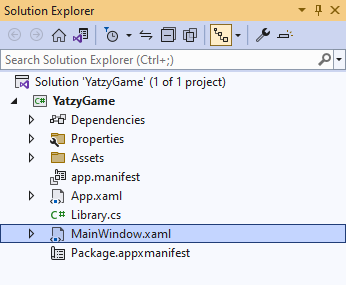## Step 27

In the XAML for MainWindow.xaml there will be some XAML for a `StackPanel`, this should be Removed:

``````
<StackPanel Orientation="Horizontal"
HorizontalAlignment="Center" VerticalAlignment="Center">
<Button x:Name="myButton" Click="myButton_Click">Click Me</Button>
</StackPanel>
``````

## Step 28

While still in the XAML for MainWindow.xaml below `<Window`, type in the following XAML:

``````
xmlns:ui="using:Comentsys.Toolkit.WindowsAppSdk"
``````

The XAML for `<Window>` should then look as follows:

``````
<Window
xmlns:ui="using:Comentsys.Toolkit.WindowsAppSdk"
x:Class="YatzyGame.MainWindow"
xmlns="http://schemas.microsoft.com/winfx/2006/xaml/presentation"
xmlns:x="http://schemas.microsoft.com/winfx/2006/xaml"
xmlns:local="using:YatzyGame"
xmlns:d="http://schemas.microsoft.com/expression/blend/2008"
xmlns:mc="http://schemas.openxmlformats.org/markup-compatibility/2006"
mc:Ignorable="d">
``````

## Step 29

While still in the XAML for MainWindow.xaml above `</Window>`, type in the following XAML:

``````
<Grid>
<Grid.Resources>
<!-- Resources -->

</Grid.Resources>
<Viewbox>
<!-- StackPanel -->

</Viewbox>
<CommandBar VerticalAlignment="Bottom">
<AppBarButton Icon="Page2" Label="New" Click="New"/>
</CommandBar>
</Grid>
``````

This XAML contains a `Grid` with a Viewbox which will Scale a `StackPanel` to be added in a later Step along with using Resources which will be added in the next Step and has an `AppBarButton` set to `New`.

## Step 30

While still in the XAML for MainWindow.xaml below the Comment of `<!-- Resources -->` type in the following XAML for Data Templates for look-and-feel of the game along with `OptionTemplateSelector`.

``````
<DataTemplate x:Name="DiceTemplate">
<StackPanel>
<ui:Dice Height="50" Width="50" Value="{Binding Value}"
<ToggleButton Margin="2" HorizontalAlignment="Center" Content="Hold"
IsChecked="{Binding Hold}" Command="{Binding Command}"
CommandParameter="{Binding}"/>
</StackPanel>
</DataTemplate>
<DataTemplate x:Key="ScoreTemplate">
<StackPanel>
<Grid Margin="2">
<Grid.ColumnDefinitions>
<ColumnDefinition Width="*"/>
<ColumnDefinition Width="Auto"/>
</Grid.ColumnDefinitions>
<Button Width="200" Grid.Column="0"
HorizontalContentAlignment="Left"
Content="{Binding Content}" Command="{Binding Command}"
CommandParameter="{Binding}"/>
<Grid Grid.Column="1" Background="Blue">
<TextBlock Width="75" Text="{Binding Score}"
TextAlignment="Center" VerticalAlignment="Center"
Foreground="WhiteSmoke"/>
</Grid>
</Grid>
</StackPanel>
</DataTemplate>
<DataTemplate x:Key="TotalTemplate">
<StackPanel>
<Grid Margin="2">
<Grid.ColumnDefinitions>
<ColumnDefinition Width="*"/>
<ColumnDefinition Width="Auto"/>
</Grid.ColumnDefinitions>
<TextBlock Width="200" Grid.Column="0" TextAlignment="Right"
Text="{Binding Content}" FontWeight="SemiBold"/>
<Grid Grid.Column="1">
<TextBlock Width="75" Text="{Binding Score}"
TextAlignment="Center" VerticalAlignment="Center"
Foreground="Blue"/>
</Grid>
</Grid>
</StackPanel>
</DataTemplate>
<ItemsPanelTemplate x:Name="ItemsTemplate">
<StackPanel Orientation="Horizontal"/>
</ItemsPanelTemplate>
<local:OptionTemplateSelector x:Key="OptionTemplateSelector"
ScoreItem="{StaticResource ScoreTemplate}"
TotalItem="{StaticResource TotalTemplate}" />
``````

## Step 31

While still in the XAML for MainWindow.xaml below the Comment of `<!-- StackPanel -->` type in the following XAML:

``````
<StackPanel Name="Display" Margin="50"
HorizontalAlignment="Center"
VerticalAlignment="Center"
<Button Margin="2" HorizontalAlignment="Stretch" VerticalAlignment="Center"
Command="{Binding Command}" CommandParameter="{Binding}" Content="Roll"/>
<ItemsControl ItemsSource="{Binding Dice}"
ItemTemplate="{StaticResource DiceTemplate}"
ItemsPanel="{StaticResource ItemsTemplate}"/>
<ItemsControl ItemsSource="{Binding Options}" HorizontalAlignment="Center">
<ItemsControl.ItemTemplate>
<DataTemplate>
<ContentControl Content="{Binding}"
ContentTemplateSelector="{StaticResource OptionTemplateSelector}"/>
</DataTemplate>
</ItemsControl.ItemTemplate>
</ItemsControl>
</StackPanel>
``````

This XAML contains a `StackPanel` with a `Loaded` event handler for `Load` it also contains a `Button` along with an `ItemsControl` for the Dice and the other Buttons and Totals for the game.

## Step 32

Within Solution Explorer for the Solution select the arrow next to MainWindow.xaml then double-click on MainWindow.xaml.cs to see the Code for the Main Window.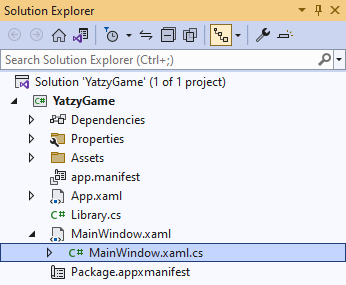## Step 33

In the Code for `MainWindow.xaml.cs` there be a Method of `myButton_Click(...)` this should be Removed by removing the following:

``````
private void myButton_Click(object sender, RoutedEventArgs e)
{
myButton.Content = "Clicked";
}
``````

## Step 34

Once `myButton_Click(...)` has been removed, within the Constructor of `public MainWindow() { ... }` and below the line of `this.InitializeComponent();` type in the following Code:

``````
private readonly Library _library = new();

private void Load(object sender, RoutedEventArgs e) =>

private void New(object sender, RoutedEventArgs e) =>
_library.New();
``````

Here an Instance of `Library` is created then below this are the Methods of `Load` and `New` that will be used with Event Handler from the `XAML`, these Methods use Arrow Syntax with the `=>` for an expression body which is useful when a Method only has one line.

## Step 35

That completes the Windows App SDK application. In Visual Studio 2022 from the Toolbar select YatzyGame (Package) to Start the application.## Step 36

Once running you can select Roll to randomly select the values for the Dice and you can use the other Buttons to total up your score based on the values of the Dice, or select New to start a new game.## Step 37

To Exit the Windows App SDK application, select the Close button from the top right of the application as that concludes this Tutorial for Windows App SDK from tutorialr.com!